JAVA, HTML5 & Chemistry Site

Other Writing

Last Update
31-Aug-2013

## Solubility in Supercritical Cabon Dioxide

2013.8.1

HSPiP Team Senior Developer, Dr. Hiroshi Yamamoto

I got the data of Solubility in Supercritical Carbon Dioxide, Ram B. Gupta, Jae-Jin Shim, CRC press.
There is one idea that the Solubility in ScCO2 is determined by melting point of the compounds. It seems to be reasonable because if melting point is high, the molecule is hard to get out.
The melting point is one specific property for one compound. The solubility data is dependent on the temperature and pressure. So, if I plot log(Solubility) to Melting point, Gupta data book result become like below.There is rough correlation, but with same MP, the solubility differ 1000000 times at large. So, at first, I choosed only Temperature(308K) and Pressure (14MPa) data and try to find the descriptors.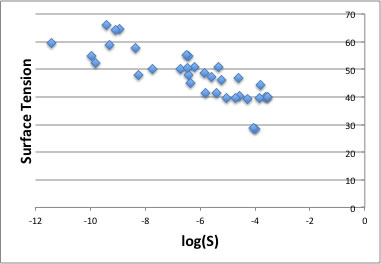For example, if I plot log(S) to Surface tension, I found very good correlation. It is very reasonable because the surface tension of ScCO2 is very low and the compound’s surface tension is low, then CO2 is easily penetrating into compounds.
And I plot log(S) to log(Viscosity) then it also has good correlation like below chart.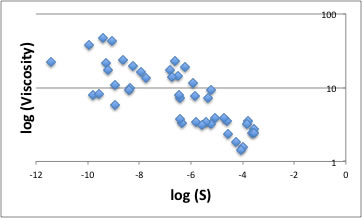You may think that the viscosity of solid (at 308K) is meaningless. But for surface tension, viscosity or other descriptors, the temperature dependent properties are all extrapolated to reference temperature even though they are solid.
Such case, the Solubility (or other properties) is dependent on surface tension and viscosity, it may also have good correlation with Marangoni number.If temperature deference(ΔT) exist on the surface, the higher temperature means low surface tension, and lower temperature means higher surface tension. Then the convection flow start. This is called marangoni flow.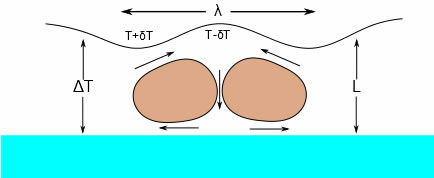(From prof. Steven Abbott’s HP)

The convection flow is determined by the Marangoni Numb.(Mg)σ: surface tension, (SI units: N/m)
L: characteristic length, (SI units: m)
α: thermal diffusivity, (SI units: m²/s)
η: dynamic viscosity, (SI units: kg/(s·m))
ΔT: temperature difference, (SI units: K)

If you want to calculate marangoni number of certain solvents at certain temperature, please use this calculator.
Here, I call Marangoni number where ΔT=1, L=1.

The larger Marangoni number it means lower viscosity and temperature dependency of surface tension is higher. Then the convection flow become strong.
This solubility result said that Marangoni flow become harder, the solubility become higher.
The sentence that Melting point has correlation with ScCO2 solubility is reasonable because the higher melting point compounds are higher surface tension like below chart.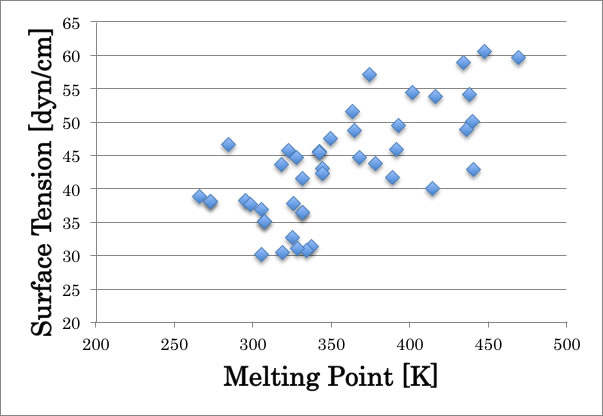The Marangoni flow is very famous phenomena in the space. But even on ground, when it come to Nano scale (depend on L or λ) like inkjet technology, Marangoni flow become important.
Inkjet, ScCO2, micelle in water, the gravity is canceled, then Marangoni flow become important.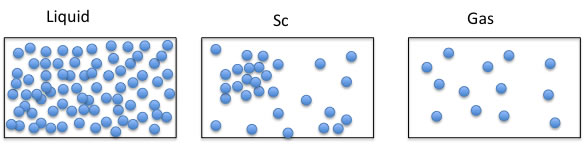In the liquid phase, the probability to impact molecules is high, but velocity of molecule is not high. In the Gas phase, velocity of molecule is very high, but seldom impact.
At the Sc(Super Critical) phase, it is something like fog. Some part, it is high density. And other part, it is low density. At the low-density part, the velocity of molecule is very high and impact to high-density part like billiard break.

What happen if the solute exists in Sc phase?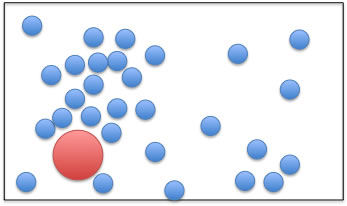The liquid solute (Red circle) contact with both high-density and low-density part of CO2. It will produce difference of surface tension and it lead to Marangoni flow.
And the strength of Marangoni flow change solubility.
So I found one interesting descriptor “Marangoni number” so I start analyzing all the range of temperature and pressure for liquid compounds (Melting point <300K). When I calculate Marangoni number, I use surface tension, thermal diffusivity and dynamic viscosity at referenced temperature. So the temperature effects are already included in Marangoni number. So the problem is pressure effect to solubility.
There is very interesting phenomena around low-pressure range.
The critical point of CO2 is Tc=31.1°C, Pc=74 bar. So almost all experiments are done over 304.25K, 74bar. But in Gupta handbook, there are some data that reference pressure is below 74 bar.

For example, Acetic acid case, the graph of convex downward as pressure increase.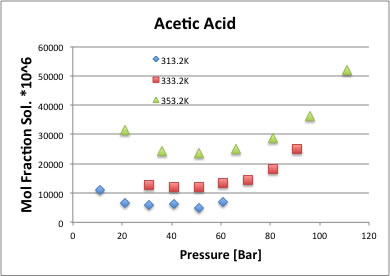The Acetone solubility is also same convex downward.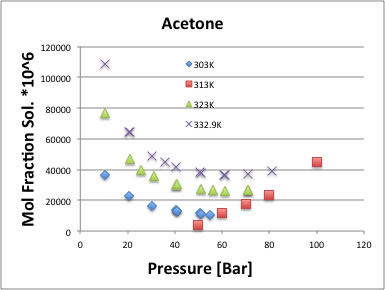It is natural that the temperature become higher, the solubility amount becomes larger. It is same with normal solvent–solution relation. But the pressure effect, that is very difficult to understand. Why the solubility decreases as increase pressure?
Why it has minimum around 50-70 bar.
Why the solubility increase after 50-70 bar.
What I found is that the reason solubility around lower pressure is high because the vapor pressure of solute is identical at one temperature, but partial pressure is decrease as CO2 pressure increasing. That means if CO2 pressure increase, the solute become hard to vaporize. So, if I estimate Antoine parameters of solutes, then I can calculate vapor pressure. Then introduce Kvp value.
Kvp=vapor pressure of solute/pressure of CO2
At first, I choose the data that the reference pressure is below 70 bar. Then plotted log(Kvp) to log solubility.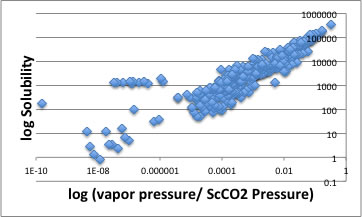There is exceptions around logS=1000. They are phthalate ester.
But almost all other solutes, CO2 pressure effect are canceled if I use Kvp parameter. So I can conclude that CO2 pressure effect to solubility is partial pressure effect. Then why the solubility increase after 50-70 bar?  The partial pressure become much smaller.
So, I make the column logS divided by Kvp=(Vapor Pressure/ScCO2 Pressure) and check which descriptor have high correlation. (Cancel Temperature and Pressure effect)

Then the highest correlated descriptor was Marangoni number.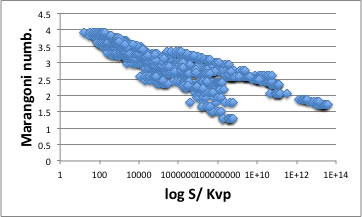I can see several threads in chart, so I divided into several categories.

Alcohol group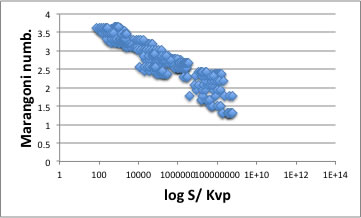Acid group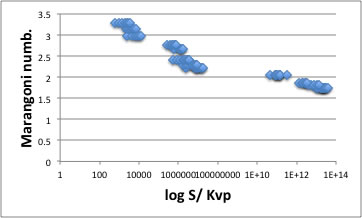Exception like phthalate ester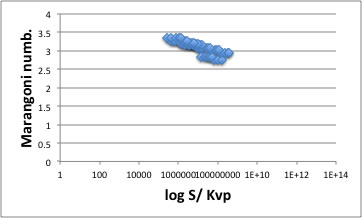Other solutes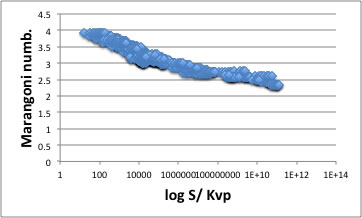And overlap all data.So, the solubility in ScCO2 is determined by Marangoni number if temperature and pressure effect are canceled.
It is still uncertain why carboxylic and alcohol compounds have different slopes of lines. That might cause from hydrogen bonding effect or low estimation accuracy of Marangoni number.
The when I say Marangoni flow, the solute should be liquid, but for the higher melting point compounds have also same tendency showed below chart. The Marangoni number became smaller and the solubility became smaller, but similar behaviors exist for each group.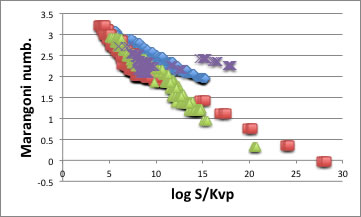I explain my thought with Benzyl alcohol (BP:478K) solubility.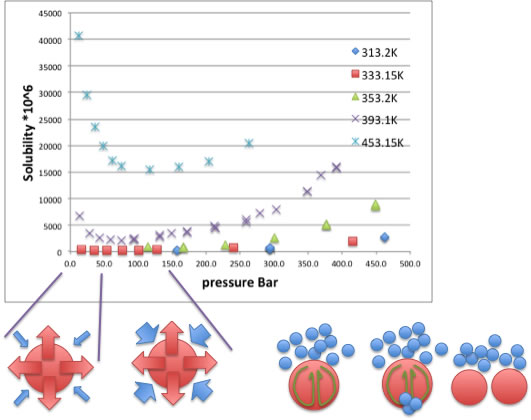The temperature is higher, vapor pressure of solute is also higher and the Marangoni flow is stronger. SO the solubility is higher as temperature increase.
When the CO2 Pressure is small, the partial pressure of solute is higher and solubility is larger. The larger CO2 pressure, the less partial pressure of solute it become. So the solubility is going down as increase pressure.
If the temperature is same, Marangoni flow is same. But the pressure of CO2 increase, CO2 begins to dissolve as flow.  That will increase of surface area and increase solubility.
I have no evidence of particle size difference as to pressure.
The result of crystallization process in ScCO2 may reflect pressure and particle size relationship.
In this ScCO2 area, they use ethanol as entrainer. It change solubility dramatically. And for polymerization in ScCO2, some fluorinated surfactant play important role. These are also indirect evidence of surface tension driven solubility.